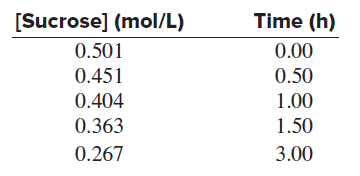⚠️Our tutors found the solution shown to be helpful for the problem you're searching for. We don't have the exact solution yet.

# Solution: The acid-catalyzed hydrolysis of sucrose occurs by the following overall reaction, whose kinetic data are given below:C12H22O11(s) + H2O(l) ⟶C6H12O6(aq) + C6H12O6(aq)     sucrose                         glucose           fructose(c) Some studies have shown that this reaction is actually second order overall but appears to follow pseudo–first-order kinetics. Suggest a reason for this apparent first-order behavior.

###### Problem

The acid-catalyzed hydrolysis of sucrose occurs by the following overall reaction, whose kinetic data are given below:

C12H22O11(s) + H2O(l) ⟶C6H12O6(aq) + C6H12O6(aq)
sucrose                         glucose           fructose(c) Some studies have shown that this reaction is actually second order overall but appears to follow pseudo–first-order kinetics. Suggest a reason for this apparent first-order behavior.